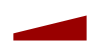Reflexxes Motion Libraries  Manual and Documentation (Type II, Version 1.2.6)
Synchronization Behavior

Contents of this page:

## Introduction

The On-Line Trajectory Generation (OTG) algorithms of the Reflexxes Motion Libraries are capable to generate

• non-synchronized,
• time-synchronized, and
• phase-synchronized

motion trajectories.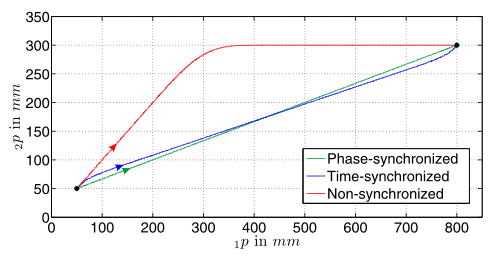Paths of non-, time-, and phase-synchronized trajectories for a two-degree-of-freedom system.

While the generation of non-synchronized and time-synchronized trajectories is always possible for both, the position-based and the velocity-based On-line Trajectory Generation algorithm, phase-synchronized trajectories require certain input values. The following image shows a non-, a time-, and phase-synchronized trajectory for three degrees of freedom.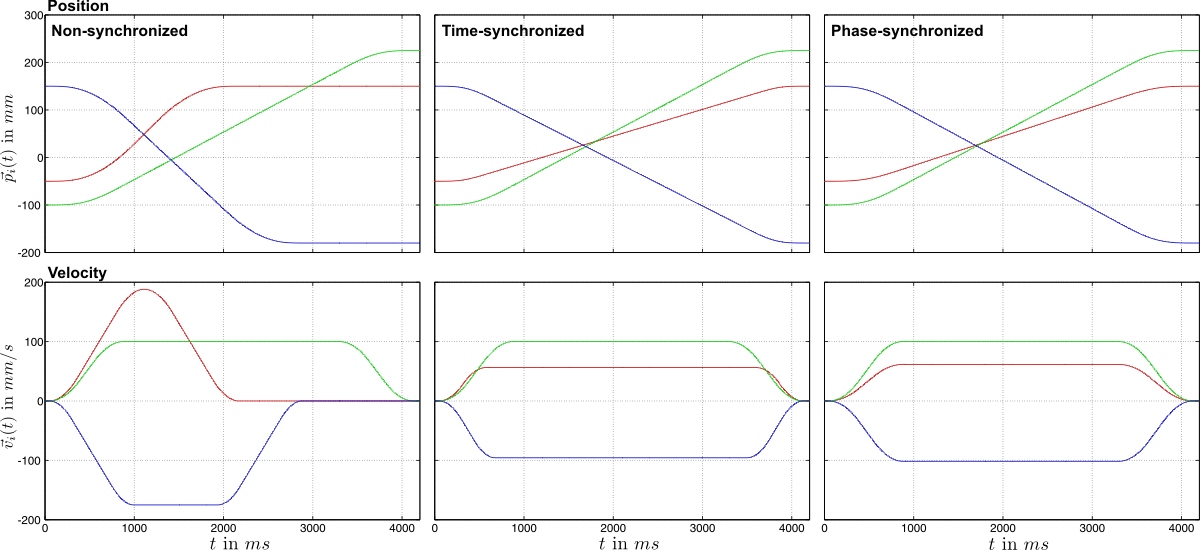Non-synchronized, time-synchronized, and phase-synchronized trajectories for a system with three degrees of freedom.

To specify the behavior of the Reflexxes Motion Library, the enumeration RMLFlags::SyncBehaviorEnum consists of four elements

and the attribute RMLFlags::SynchronizationBehavior is used to specify the synchronization behavior of the desired trajectory.

Detailed information about the flag RMLFlags::PHASE_SYNCHRONIZATION_IF_POSSIBLE can be found on page About the Flag RMLFlags::PHASE_SYNCHRONIZATION_IF_POSSIBLE.

## Synchronization Behavior of the Position-based On-line Trajectory Generation Algorithm

All four values of the set RMLFlags::SyncBehaviorEnum can be used to specify the synchronization behavior of the position-based On-Line Trajectory Generation algorithm (cf. ReflexxesAPI::RMLPosition()).

An example showing, how to set-up time- and phase-synchronized motion trajectories, is shown at page Example 3 — Different Synchronization Behaviors of the Position-based algorithm. The resulting time- and phase-synchronized trajectories and paths of the two degrees of freedom of this example are depicted in the following figure.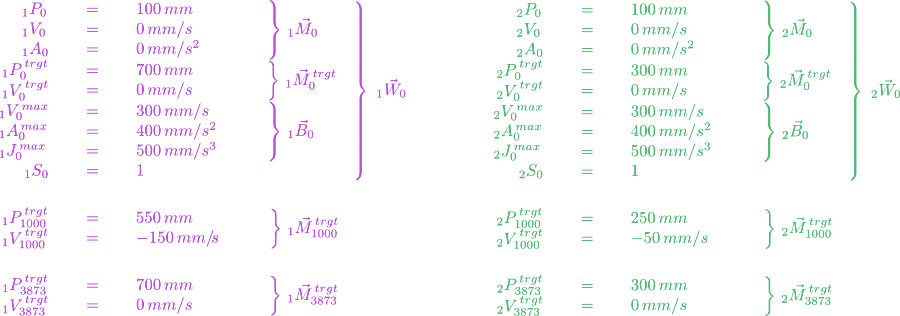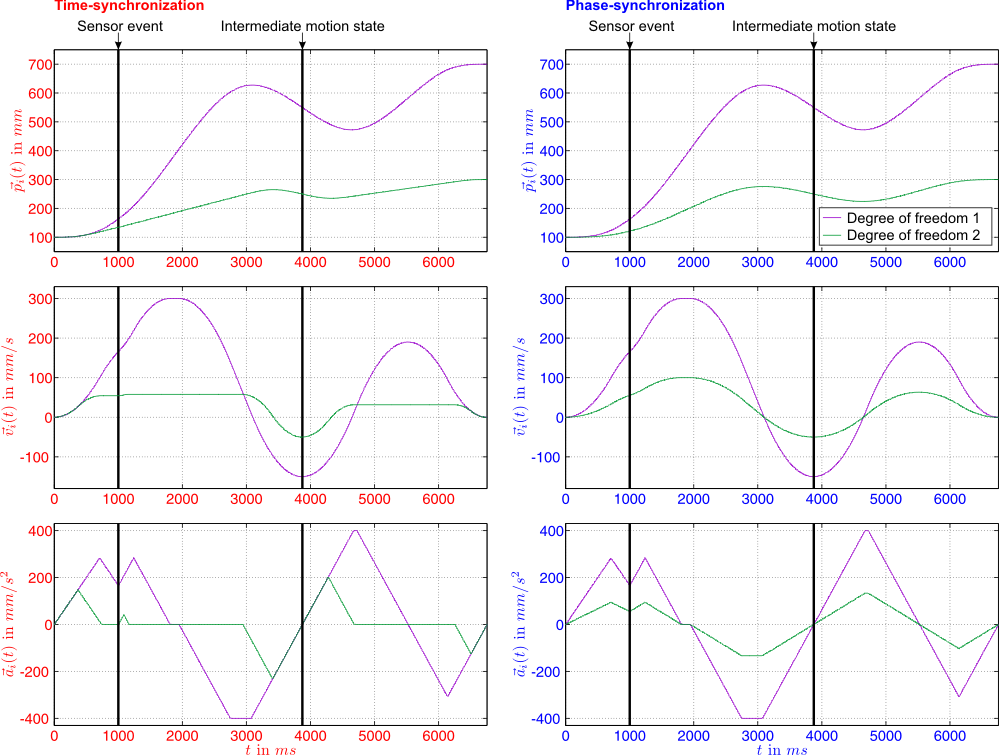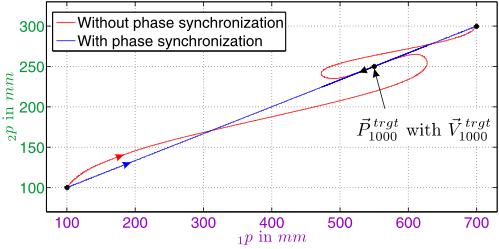Example of a two-degree-of-freedom time- and phase-synchronized motion trajectory (top) and corresponding paths (bottom) generated by the position-based Type IV On-Line Trajectory Generation algorithm of the Reflexxes Motion Libraries using the same input values (Type IV).

In this example, the task is to execute a motion from standstill, which is defined by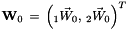. After 1000 ms, a sensor event happens (unforeseen), and due to this, we have to immediately react and now have to reach a position ahead with a negative velocity. This desired state of motion,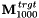, is time-optimally reached after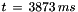(cf. figure above), and right after this, a further switching of set-points happens, such that the originally desired state of motion,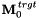, shall be reached again. After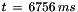, this desired state is finally reached (cf. Example 3 — Different Synchronization Behaviors of the Position-based algorithm).

## Synchronization Behavior of the Velocity-based On-line Trajectory Generation Algorithm

The velocity-based On-Line Trajectory Generation algorithm (cf. ReflexxesAPI::RMLVelocity()) can also generate

• non-synchronized,
• time-synchronized, or
• phase-synchronized,

and accepts any values of RMLFlags::SyncBehaviorEnum. The default value for the corresponding flag RMLVelocityFlags::SynchronizationBehavior is RMLFlags::NO_SYNCHRONIZATION. The following image shows an example for a non-synchronized and a time-synchronized trajectory using the same input values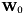.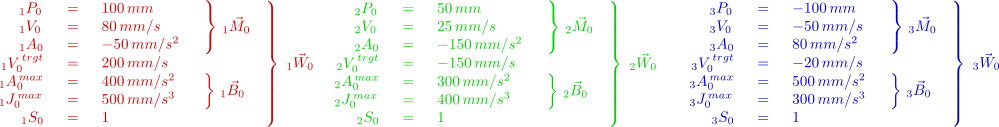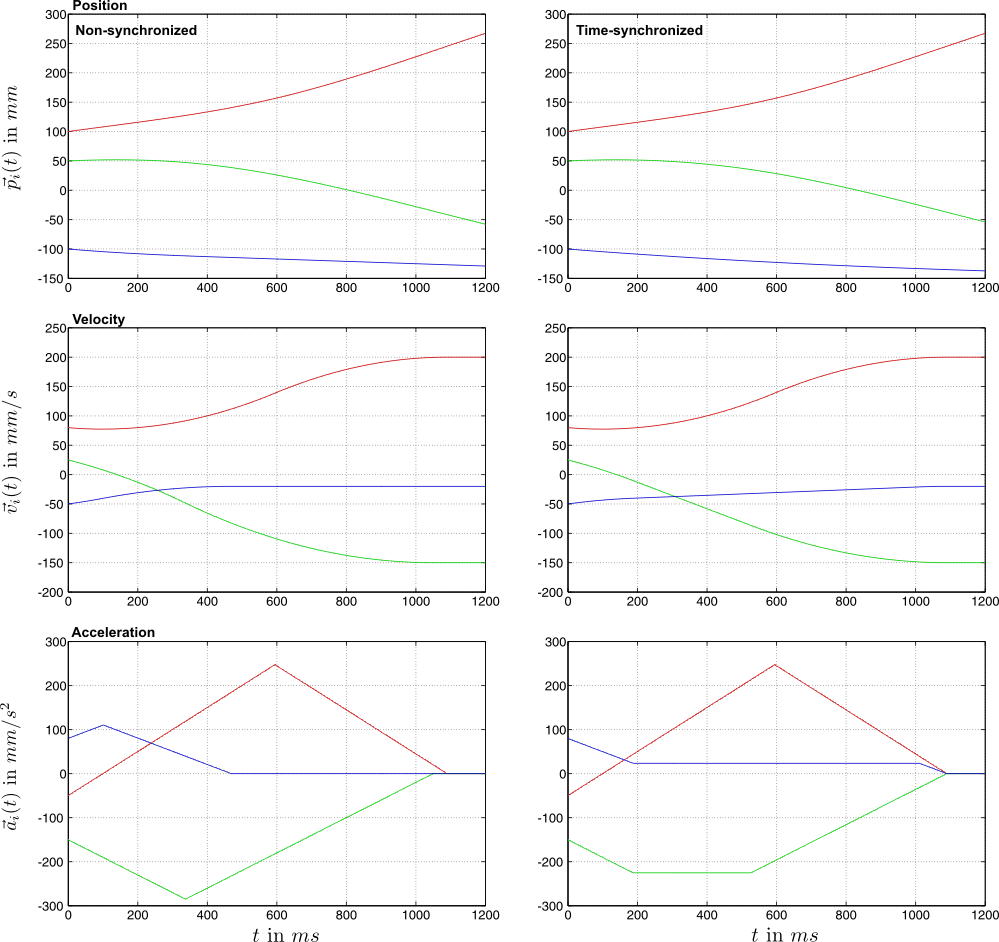Example of a Three-degree-of-freedom trajectory generated by the velocity-based On-line Trajectory Generation algorithm. The left trajectory is non-synchronized;the right one is time-synchronized (Type IV).

Note:
The examples above have been generated with the Type IV Reflexxes Motion Library, which takes into account the values of

User documentation of the Reflexxes Motion Libraries by Reflexxes GmbH (Company Information, Impressum). This document was generated with Doxygen on Mon Jul 7 2014 13:21:09. Copyright 2010–2014.